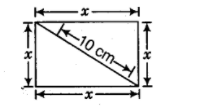# Find the length of the side of a square,

Question:

Find the length of the side of a square, if the length of its diagonal is 10 cm.

Solution:

Given, length of diagonal $=10 \mathrm{~cm}$

Suppose, the length of side of a square is $x \mathrm{~cm}$.

By using Pythagoras theorem,$(10)^{2}=x^{2}+x^{2}$

$\Rightarrow \quad 100=2 x^{2}$

$\Rightarrow \quad x^{2}=50$

$\Rightarrow \quad x=\sqrt{50}$  [taking square root on both sides]

$\therefore \quad x=5 \sqrt{2} \mathrm{~cm}$

Hence, the length of the side of square is $\sqrt{50}$ or $5 \sqrt{2} \mathrm{~cm}$.